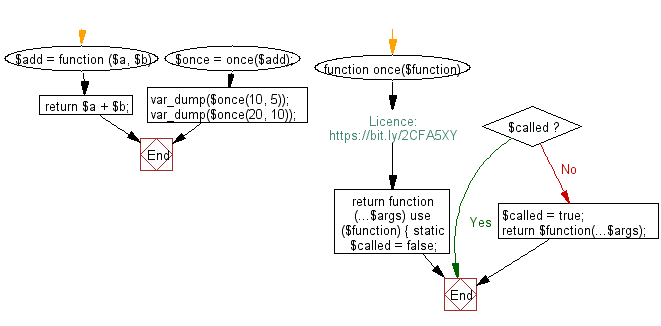﻿ PHP Exercise: Call a given function only once - w3resource

# PHP Exercises: Call a given function only once

## PHP: Exercise-101 with Solution

Write a PHP program to call a given function only once.

Sample Solution: -

PHP Code:

``````<?php
//Licence: https://bit.ly/2CFA5XY

function once(\$function)
{
return function (...\$args) use (\$function) {
static \$called = false;
if (\$called) {
return;
}
\$called = true;
return \$function(...\$args);
};
}

\$add = function (\$a, \$b) {
return \$a + \$b;
};

var_dump(\$once(10, 5));
var_dump(\$once(20, 10));

?>
```
```

Sample Output:

```int(15)
NULL
```

Flowchart:PHP Code Editor:

Have another way to solve this solution? Contribute your code (and comments) through Disqus.

What is the difficulty level of this exercise?

Test your Programming skills with w3resource's quiz.

﻿

## PHP: Tips of the Day

Returns all elements in an array except for the first one

Example:

```<?php
function tips_tail(\$items)
{
return count(\$items) > 1 ? array_slice(\$items, 1) : \$items;
}

print_r(tips_tail([1, 5, 7]));
?>
```

Output:

```Array
(
 => 5
 => 7
)
```